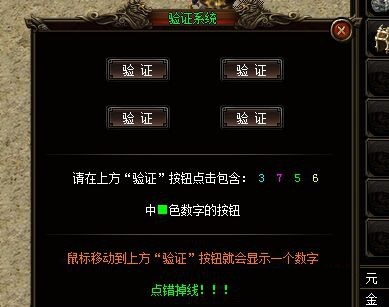# 七星辅助A版过gee引擎验证按钮点击数字按钮脚本这是单职业gee引擎闲云传的防脱机验证码，这个验证对于七星辅助来说也能过掉，只不过目前只能开启七星辅助A版，后续如果七星辅助B版能开再给大家补上b的过验证脚本，七星辅助A版过请在上方验证按钮点击包含什么色数字的按钮防脱机验证脚本如下：

新增B版过验证码脚本：定时脚本执行

function 过随机验证（）
local a=获取NPC对话内容（）
if string.find（a,"@掉线处理"）~=nil then
i=0
j=0
while true do
i,j,cmd=string.find（a,"（@.-）>",j）
if cmd~="@掉线处理" then
break
end
end
选择（cmd）
等待（1500）
end
return 2
end

function 回收（）
return 挂机（）
end

function 挂机（）
清空挂机路线（）
开始挂机（）
return 回收（）
end

do
--开始
设置用户检测（"过随机验证",2000）
挂机（）
end

--B版定时脚本

local a=获取NPC对话内容（）
if string.find（a,"@掉线处理"）~=nil then
i=0
j=0
while true do
i,j,cmd=string.find（a,"（@.-）>",j）
if cmd~="@掉线处理" then
break
end
end
选择（cmd）
等待（1500）
清空NPC对话内容（）
end

85%的户主都看了以下辅助验证码下载